## "Sample Size Planning for Estimating Prevalence of Sensitive Attributes Using a Non-Randomized Response Model"

Guangyong Zou*

Department of Epidemiology & Biostatistics, University of Western Ontario, Canada

*Corresponding author: Guangyong Zou, Department of Epidemiology & Biostatistics, University of Western Ontario, Canada. Tel: +15196612111; Ext: 86298; Fax: +15196613766; Email: gy.zou@robartsinc.com
Received Date: 20 July, 2018; Accepted Date: 13 August, 2018; Published Date: 20 August, 2018

1. Abstract

It is well known that prevalence of a sensitive attribute may be underestimated based on direct inquiry of subjects. A non-randomized response model has thus been proposed and shown to be efﬁcient in estimating the prevalence of sensitive attributes in surveys. Since most surveys are conducted to obtain precise estimates, herein we derive a sample size formula for this model based on conﬁdence interval estimation rather than hypothesis testing as estimation is of most relevance in this context. In contrast to the conventional approach to sample size estimation, which does not explicitly consider the chance of achieving the precision, we incorporate an assurance probability into the formula by treating conﬁdence interval width as random. Exact evaluation demonstrates that our formula performs well.

1. Keywords: Conﬁdence Interval; Prevalence; Proportion; Random Response

3.Introduction

Reliable information about prevalence of attributes is crucial to the success of many public health preventions and interventions. Oftentimes, one may need to estimate prevalence of sensitive attributes such as illicit drug use, risky behavior, cheating, or non-adherence to prescribed medication or treatment. Directly asking people about such sensitive questions is generally problematic because of refusal or response bias. To overcome such difficulty, much effort has been made to develop methods that are effective in obtaining reliable information on sensitive attributes.

Warner  proposed a method that is commonly known as the random response design, which is very convenient to use in face-to-fact interviews. Specifically, an interviewee is presented with two mutually exclusive statements about the sensitive attributes, such as (a) ‘I cheated’ and (b) ‘I never cheated’ and is then instructed to provide an answer of ‘Correct’ or ‘Incorrect’ for statement (a) or (b), depending on the outcome from random device such as a dice or spinner provided by the interviewer. Without the interviewer knowing the outcome of the random device, the interviewee then provided an answer. The privacy of the interviewee is protected by the fact that the interviewer only knows the answer but does not know to which statement the interviewee is referring. While the outcome of the random device for every individual is unknown to the interviewer, the chance of the random device directing the interviewee to answer (a) and (b) is controlled by the interviewer. Estimation of the prevalence of the sensitive attributes can then be made at the aggregate level, using maximum likelihood theory .

The Warner model has been used in a wide range of contexts as reviewed in Lensvelt-Mulders, et al. . However, previous research has shown that the Warner model has several drawbacks. First, the technique is limited to face-to-face interviews, thus cannot be used in other cases such as mail surveys. Second, it can be difﬁcult to convince a respondent that privacy has actually been protected by the random device, since the chance of providing an answer to the sensitive statement is controlled by the interviewer. Finally, the estimate can be very inefﬁcient as compared to direct questioning approach.

To overcome these limitations Yu, et al.  has proposed a non-randomized model that uses an independent non-sensitive statement such as season of birth in the survey to indirectly obtain the answer to the sensitive question. For example, to estimate the prevalence of cheating, a respondent is asked to answer a ‘Correct’ or ‘Incorrect’ to the following statement: ‘I have never cheated and my mother was born between May and August’. Previous research has shown that this non-randomized model can be a viable alternative to the Warner random response model .

The purpose of this paper is to derive corresponding sample size formula for estimating the prevalence of sensitive attributes. In contrast to that for hypothesis testing , our focus is on conﬁdence interval estimation, which usually is the objective of most surveys. Conventional sample size formula for estimation is derived based on the expected conﬁdence interval width, thus a survey so designed can only have about 50% chance of achieving the desired precision . Therefore, our objective is to derive a sample size formula for conﬁdence interval construction that incorporates assurance probability of achieving the desired interval width.

The rest of the paper is organized as follows. Section 2 provides a brief review of a non-randomized design . Sample size formula is derived and illustrated in Section 3, followed by an evaluation of the performance in Section 4. The paper closes with a summary.

1. A Nonrandomized Triangular Design for Sensitive Attributes

Let Y denote the variable of the sensitive attribute of interest, such as cheating, with value 1 being ‘have cheated’ and 0 not cheated. Let W be a non-sensitive binary attribute that is independent of Y, such as ‘born between May and August’. The W here should be so chosen that the probability of W = 1 is known or easily estimated. Denote Pr(W = 1) by p. The aim is to estimate the probability of Y = 1, denoted as π.

In a face-to-face interview, the interviewer may use the format on the left-hand side of Table 1 and ask the interview to put a tick in the open circle or in the triangle formed by the three dots, depending on whether or not the event {Y = 0 and W = 0} is true. This design has been referred to as the triangular design by Yu, et al. . The cell probabilities for the right side of Table 1 can be obtained by multiplying the marginal probabilities, since W and Y are independent by design. Thus, the probability of ticking the circle is given by (1 - π)(1 - p), while that for ticking the triangular is 1 - (1 - π)(1 - p), i.e., π + (1 - π)p, where p is assumed to be a known constant. Thus, the number of respondents ticking the triangular follows a binomial with parameters of n and π + (1 − π)p. Denoting Δ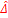the proportion of respondents ticking the triangular, we have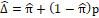which yields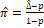with variance given by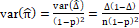(1)

which may be consistently estimated by substituting ∆̂ for ∆. A (1 - α)100% two-sided conﬁdence interval for π can thus be obtained as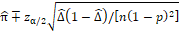(2)

where and throughout the paper zα/2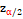is the upper α/2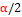quantile of the standard normal distribution. Alternatively, one can ﬁrst apply the Wilson  method for constructing a conﬁdence interval for , and then obtain a conﬁdence interval for π as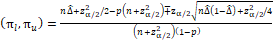(3)

Previous results have shown that the Wilson method performs very well [8,9], which in turn suggests that this interval may perform better than the one in Equation (2) when sample size is not very large and the true prevalence of the attribute is low.

1. Derivation of the Sample Size Formula

Now, suppose an investigator wish to estimate the prevalence of a sensitive attribute using the non-randomized triangular design. To ensure the survey results in a precision estimate of π, she speciﬁes that the half width of the two-sided (1 - α)100% conﬁdence interval for π to be at most ω with a probability of (1 - β)100%. This probability is referred to as the assurance probability since it quantifies how likely the precision of the estimate will be achieved 

Since the half width based on Equation (2) is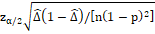the requirement can then be written as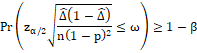or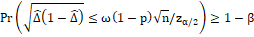(4)

Thus, we need to ﬁnd the asymptotic distribution for  Δ1-Δ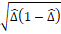. ). By the delta method, we have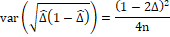By the central limit theorem,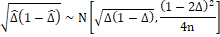Applying this result to equation (4), we have asymptotically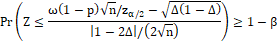i.e.,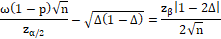Solving for n and squaring the admissible solution, we have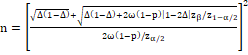(5)

which reduces to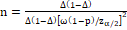when β = 0.5.

When p = 0, i.e., asking the question directly, the formula in Equation (5) reduces to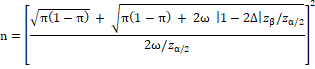which, for β = 0.5, further reduces to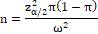the formula for estimating a proportion appeared in most text books. Note also that for a given sample size n, we can obtain the assurance probability 1 - β, because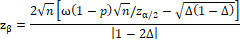To illustrate sample size formula (5), consider the example used by Tian, et al. . A survey used the triangular non-randomized design to estimate proportion of women aged 20 to 44 who have undergone induced abortion in the South district of Taichung City in Taiwan. With p = 0.3, the estimated proportion is π = 0.25, with a 95% conﬁdence interval of 0.05 to 0.45. Using this information, an investigator wishes to have 90% chance that the half width of the 95% conﬁdence interval is no greater than 25% of the point estimate, i.e. ω = 0.0625. Since, = 0.25 + (1 − 0.25) × 0.3 = 0.475. Using Equation (5), we obtain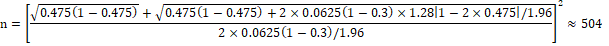as the required sample size in this case.

1. Evaluation of the Sample Size Formula

To assess the accuracy of the sample size formulation in Equation (5), we performed an evaluation based on the binomial distribution. We considered parameter combinations of π = 0.05, 0.1, 0.3, 0.5, p = 0, 0.3, 0.5, half width ω of 95% conﬁdence interval as 25% and 50% of π, and assurance probability 1 - β of 50% and 95%. For given values of p, π (thus ), ω, and 1 - β, we ﬁrst calculated sample size n required for a 95% conﬁdence interval using the formula in Equation (5). We then obtained the 95% conﬁdence interval (l,u) for π using the formula in Equation (3). Finally, we calculated the empirical assurance probability as n

Empirical Assurance =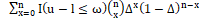where I (·) is the indicator function that takes value 1 if the condition is satisﬁed, and 0 otherwise. We also consider empirical coverage percentage of the coverage intervals as follows

Empirical Coverage =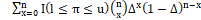the left and right tail errors are deﬁned analogously.

Results in Table 2 show that the empirical assurance probabilities are above the nominal level, especially for the 95% level. The conﬁdence interval procedure performs well in terms of overall coverage and balance of tail errors.

1. Conclusion

It has been well-known that sample size estimation based on expected conﬁdence interval width can provide very low assurance in achieving the desired precision . Although sample size calculation incorporating assurance probabilities for conﬁdence interval estimation of normal means and their differences have been provided by Kupper and Hafner  and Beal , these results have not widely penetrated to statistical practice. We believe this is partly because of lack of closed form formula.

We have presented a simple formula for calculating sample size for the non-randomized triangular design when the objective is to estimate the prevalence of sensitive attributes. In contrast to conventional sample size determination for conﬁdence interval estimation, our formula incorporates an assurance probability of achieving the desired conﬁdence interval width. Similar idea has been applied to the estimation of intraclass correlation coefficient in the context of reliability studies . For simplicity, we derived the sample size formula on the basis of the Wald conﬁdence interval. The evaluation results show that the formula perform well for the Wilson-type conﬁdence interval in a wide range of parameter combinations. Further results regarding sample size calculations for a difference between two proportions in this context can be found in Qiu, et al. .

 Category W=0 W=1 Category W=0 W=1 Total Y=0 ⃝ ∙ Y=0 1-π1-p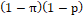1-πp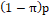1-π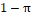Y=1 ∙ ∙ Y=1 π1-p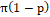πp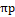π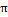Total 1-p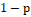p1 Respondent: Please truthfully put a tick in the circle or in the triangle formed by the three dots. Please answer ‘Correct’ if the following statement is true; otherwise answer ‘Incorrect’: I have never cheated and I was born between May and August.

Table 1: The triangular nonrandomized model and the corresponding marginal probabilities

 50% Assurance 95% Assurance π ω† n EA‡ CV ¥(L,U) n EA CV(L,U) p=0.5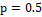0.05 25 24524 59.90 95.03 (2.48, 2.49) 24544 95.67 94.95 (2.52, 2.53) 50 6131 69.80 94.96 (2.56, 2.48) 6142 99.94 95.09 (2.45, 2.46) 0.1 25 6085 60.43 94.98 (2.48, 2.54) 6150 95.81 94.94 (2.50, 2.56) 50 1522 74.20 94.97 (2.65, 2.38) 1532 99.90 94.90 (2.53, 2.56) 0.3 25 622 59.46 95.18 (2.33, 2.48) 642 95.67 94.82 (2.51, 2.67) 50 156 74.51 95.64 (2.40, 1.96) 166 99.84 94.92 (2.29, 2.79) 0.5 25 185 58.93 94.93 (3.02, 2.05) 204 95.31 95.72 (2.36, 1.92) 50 47 56 72.82 95.74 (3.02, 1.24) 56 99.74 95.68 (2.59, 1.73) p=0.3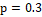0.05 25 11178 52.34 95.05 (2.44, 2.51) 11273 91.57 94.95 (2.51, 2.54) 50 2795 55.15 95.05 (2.48, 2.47) 2842 92.58 95.09 (2.40, 2.51) 0.1 25 2924 55.58 95.15 (2.42, 2.43) 2962 93.36 95.00 (2.49, 2.51) 50 731 59.30 95.37 (2.27, 2.36) 750 96.47 95.08 (2.40, 2.51) 0.3 25 349 100 95.26 (2.38, 2.36) 350 100 94.59 (2.71, 2.70) 50 88 100 95.78 (2.25, 1.97) 88 100 95.78 (2.25, 1.97) 0.5 25 115 79.78 94.98 (2.39, 2.63) 123 100 95.34 (2.54, 2.12) 50 29 100 94.90 (2.06, 3.03) 33 100 93.40 (3.77, 2.83) p=0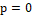0.05 25 1168 45.98 94.84 (1.92, 3.24) 1343 90.05 94.80 (2.17, 3.03) 50 292 39.83 94.17 (2.02, 3.80) 377 90.54 95.69 (1.76, 2.55) 0.1 25 554 51.32 95.31 (2.11, 2.58) 631 91.40 94.60 (2.31, 3.08) 50 139 47.00 95.17 (1.17, 3.66) 177 92.26 95.57 (1.40, 3.02) 0.3 25 144 66.57 94.41 (2.35, 3.24) 157 98.31 95.52 (1.96, 2.52) 50 36 83.74 95.65 (2.16, 2.19) 43 100 93.46 (3.11, 3.42) 0.5 25 62 100 94.41 (2.79, 2.79) 62 100 94.41 (2.79, 2.79) 50 16 100 92.32 (3.84, 3.84) 16 100 92.32 (3.84, 3.84) ω†: Half width of confidence interval as given by the percentage of p. EA‡: Empirical assurance probability.  CV ¥: coverage percentage; L: missing the parameter value from its left; R: missing the parameter value from its right.

Table 2: Performance of the sample size formula based on exact evaluation.

Citation: Zou GY (2018) Sample Size Planning for Estimating Prevalence of Sensitive Attributes Using a Non-Randomized Response Model. Arch Epidemiol: AEPD-119. DOI:10.29011/2577-2252.100019